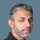cancel
Showing results for
Did you mean:Anonymous
Not applicable

## How to select Max date in a "between" type date slicer

Hi,

I am using "between" type slicer for dates to filter two tables. one is for day basis data and another is for MTD.

In Between Slicer, i'm selecting dates 1st to 9th of September.

In MTD table, it is working fine as there i need data of 1st to 9th of September.(This is completed)

Another table needs to filter only for last date which is 9th of september in this case.

How to solve this.

Need help.
Thanks,
Gaurav

2 ACCEPTED SOLUTIONSSuper User

@Anonymous , Try like

measure =
var _max =maxx(allselected(date), Date[date])
return
calculate([measure], filter(Date, Date[date] =_max))Community Support

Hi @Anonymous ,

You can use the following filter in your measure(Use date column in Date table for slicer). For MTD table:

``FILTER(ALL(MTD TABLE),MTD TABE[Date] IN VALUES(Date[Date]))``

For another table:

``FILTER(ALL(ANOTHER TABLE),ANOTHER TABE[Date]= MAX(Date[Date]))``

If this post helps, then please consider Accept it as the solution to help the other members find it more quickly.

Best Regards,

Dedmon Dai

4 REPLIES 4Super User

@Anonymous , Try like

measure =
var _max =maxx(allselected(date), Date[date])
return
calculate([measure], filter(Date, Date[date] =_max))Anonymous
Not applicable

Hi @

measure =
var _max =maxx(allselected(date), Date[date])
return
calculate([measure], filter(Date, Date[date] =_max))

This measure is the Calculated measure used or above measure.If this is calculated measure then how i'll solve the same for 5 measure and one count(value) coming from table.Community Support

Hi @Anonymous ,

You can use the following filter in your measure(Use date column in Date table for slicer). For MTD table:

``FILTER(ALL(MTD TABLE),MTD TABE[Date] IN VALUES(Date[Date]))``

For another table:

``FILTER(ALL(ANOTHER TABLE),ANOTHER TABE[Date]= MAX(Date[Date]))``

If this post helps, then please consider Accept it as the solution to help the other members find it more quickly.

Best Regards,

Dedmon DaiSuper User

@Anonymous

You can get the last date using te following function and use it in your formula.

_LastDate = MAX(Dates[Date])

________________________

If my answer was helpful, please consider Accept it as the solution to help the other members find it

Click on the Thumbs-Up icon if you like this reply 🙂

Did I answer your question? Mark my post as a solution! and hit thumbs up